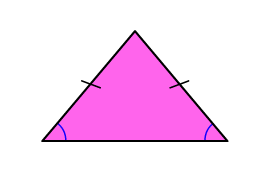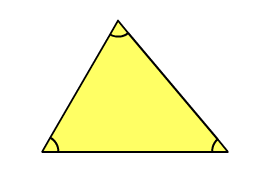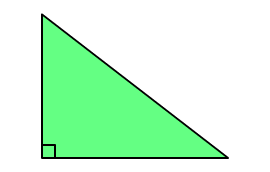# Classify Triangles

Rate 0 stars Common Core
Quiz size:
Message preview:
Someone you know has shared Classify Triangles quiz with you:

To play this quiz, click on the link below:

https://www.turtlediary.com/quiz/classify-triangles.html

Hope you have a good experience with this site and recommend to your friends too.

Login to rate activities and track progress.
Login to rate activities and track progress.

 Scalene triangleAll three sides are of different lengths All three angles are of different measures Isosceles triangleTwo sides are of same length Two angles are of same measure Equilateral triangleAll three sides are of same length All three angles are of same measure Acute triangleAll three angles are less than 90° Right triangleOne angle is exactly 90° Obtuse triangleOne angle is more than 90°

ds

A B C D E F G H I J K L M N O P Q R S T U V W X Y Z

### Help

##### Remember :

The smallest number is the one that comes first while counting.

##### Solution :

To arrange the given numbers in order from smallest to greatest, find the smallest number among all the given numbers.

21,27,23

21 is the smallest number.